## Exercise 1 - Customising simple plots

Plotting a baby’s growth rate.

``````read.delim("weight_chart.txt") -> weight.chart
weight.chart``````

We’re going to use a normal scatterplot to plot the two values against each other. We’ll add a bunch of customisation by modifying the parameters we pass.

``````plot(
weight.chart\$Age,
weight.chart\$Weight,
type="b",
pch=15,
cex=1.5,
lwd=2,
ylim=c(2,10),
xlab="Age (months)",
ylab="Weight (kg)",
main="Weigh gain during early infant development"
)``````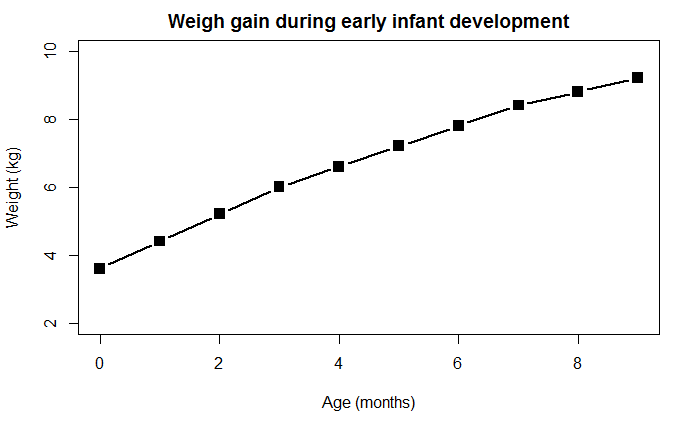Now we can read in the feature counts data to plot a barplot of the counts for different types of features.

``````read.delim("feature_counts.txt") -> feature.counts
feature.counts``````

We’re going to draw a customised barplot from this data.

``````par(mar=c(5,12,4,2))
barplot(
feature.counts\$Count,
horiz = TRUE,
xlab="Number of feature instances",
names.arg=feature.counts\$Feature,
main="Number of different feature types found",
las=1
)``````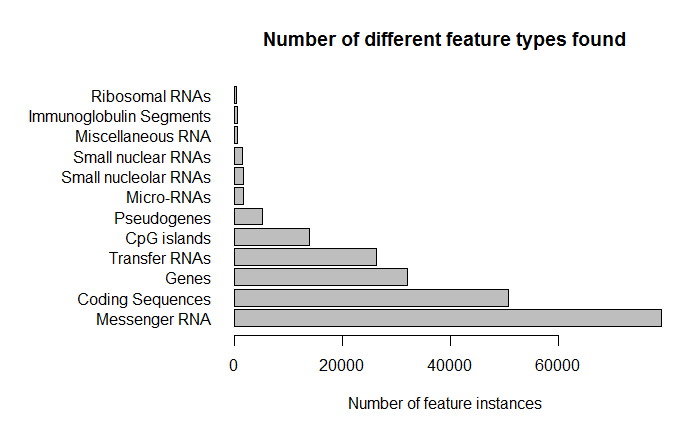Finally we want to make a biased random dataset which we can plot out as a histogram.

``````hist.data <- c(rnorm(10000),rnorm(10000)+4)
hist(hist.data,breaks=60,main="Bimodal data")``````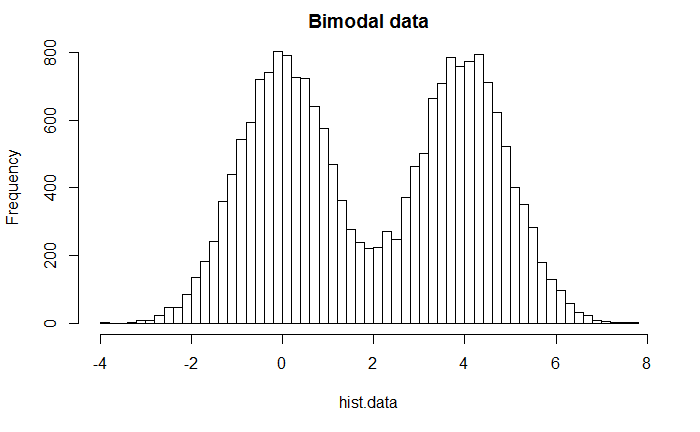## Exercise 2 - Using colour

We want to plot out the male/female count data as a barplot and change the colour in different ways.

``````read.delim("male_female_counts.txt") -> male.female
male.female``````

Now we can plot this. The barplot just needs the count data, and we’ll specifically need to add the sample names as labels. We need to make the labels small so they all fit (we could also have turned the plot around the other way).

``````barplot(
male.female\$Count,
names.arg = male.female\$Sample,
cex.names = 0.5
)``````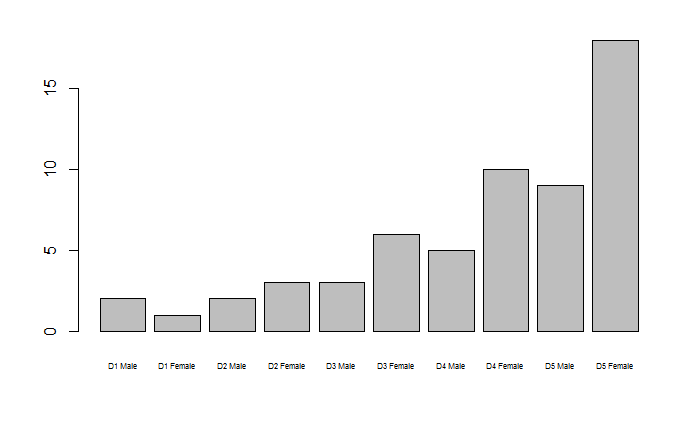To add a different colour to each point (which isn’t generally a great idea!) we can use the rainbow function to generate a set of colours. We simply need to tell the function how many colours to generate, which in this case is the number of rows in the data frame.

``rainbow(nrow(male.female))``
``````  "#FF0000FF" "#FF9900FF" "#CCFF00FF" "#33FF00FF" "#00FF66FF" "#00FFFFFF" "#0066FFFF"
 "#3300FFFF" "#CC00FFFF" "#FF0099FF"``````

We can then pass this vector as the col paramter when drawing the barplot.

``````barplot(
male.female\$Count,
names.arg = male.female\$Sample,
cex.names = 0.5,
col=rainbow(nrow(male.female))
)``````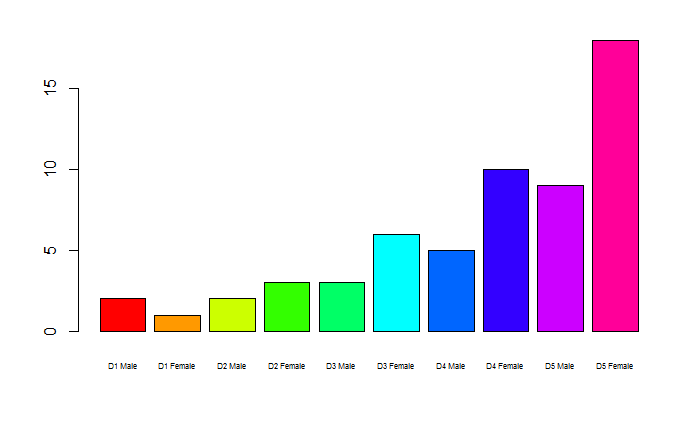Now we want to make the males and females different colours. We can’t just pass the Sample column as colour since all of the sample names are different (even though they contain Male and Female). In this specific case, because males and females alternate we can just pass a fixed 2 colour vector.

``````barplot(
male.female\$Count,
names.arg = male.female\$Sample,
cex.names = 0.5,
col=c("blue2","red2")
)``````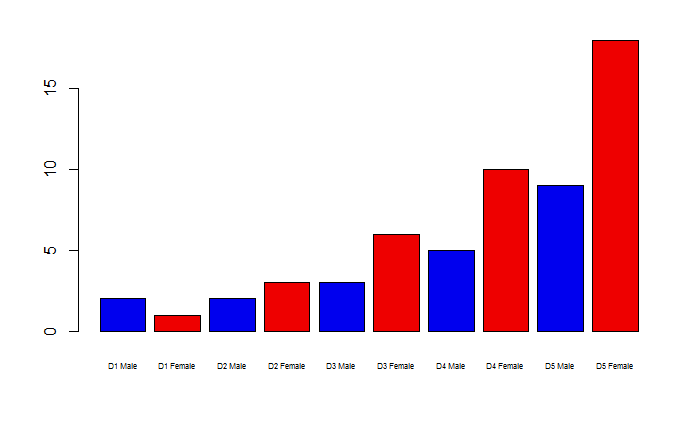If we’d wanted a more generic solution then we’d need to use some of the text mainipulation techniques shown in the advanced R course, specifically we can remove the D1 etc from the start of each string to leave us with a Male/Female split which we can use as a colour directly.

``substring(male.female\$Sample,4)``
``  "Male"   "Female" "Male"   "Female" "Male"   "Female" "Male"   "Female" "Male"   "Female"``
``````barplot(
male.female\$Count,
names.arg = male.female\$Sample,
cex.names = 0.5,
col=as.factor(substring(male.female\$Sample,4))
)``````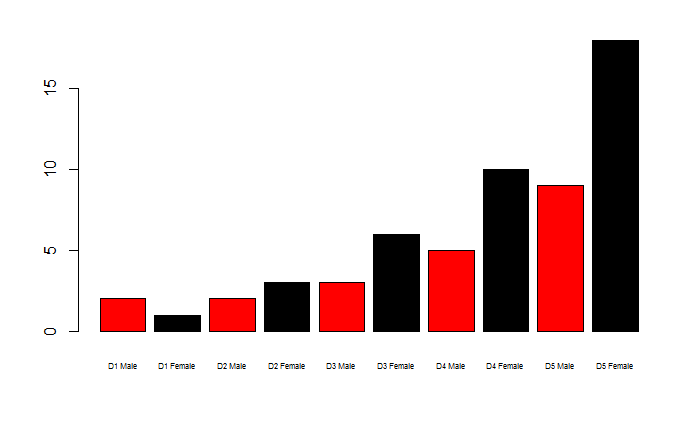We next want to use this type of categorical colour definition to highlight specific points in a scatteprlot. We can start by reading in the data.

``````read.delim("up_down_expression.txt") -> up.down
up.down``````

You can see that the data to plot are the Condition1 and Condition2 columns, and the categories are in the State column.

We can start by doing a simple uncoloured plot.

``````plot(
up.down\$Condition1,
up.down\$Condition2,
pch=19
)``````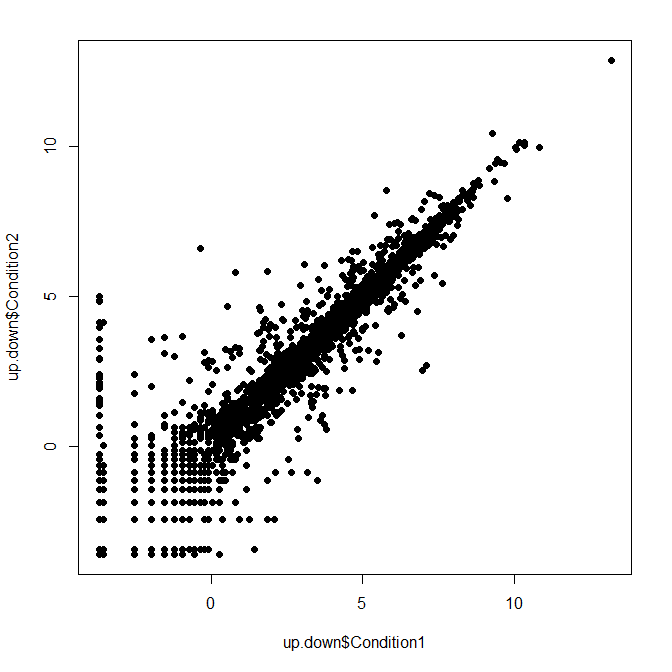Now we can colour by the State column.

``````plot(
up.down\$Condition1,
up.down\$Condition2,
pch=19,
col=up.down\$State
)``````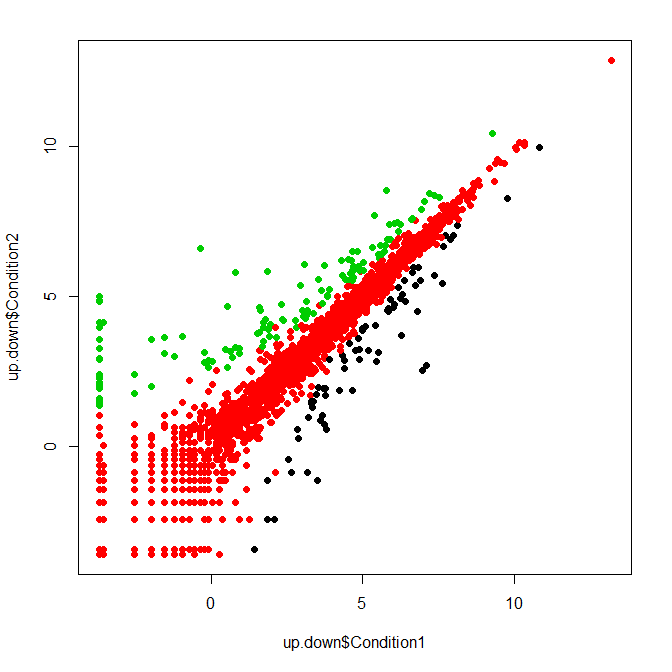We can see that 3 different groups have been coloured, but we need to understand how. We can figure this out by looking at the levels of the state column and the colour vector contained in the current system palette.

``````palette()
``````
`` "black"   "red"     "green3"  "blue"    "cyan"    "magenta" "yellow"  "gray"   ``

We can see that there is a direct mapping between the two vectors where down=black (position 1), unchanging=red and up=green. If we want to use our own colours then we need to set the palette to our colour choice in the order down,unchanging,up. In this case I’ve also added a legend so we can see which colour is which.

``````palette(c("blue2","grey","red2"))
plot(
up.down\$Condition1,
up.down\$Condition2,
pch=19,
col=up.down\$State
)
legend("topleft",levels(up.down\$State),fill=palette()[1:3])``````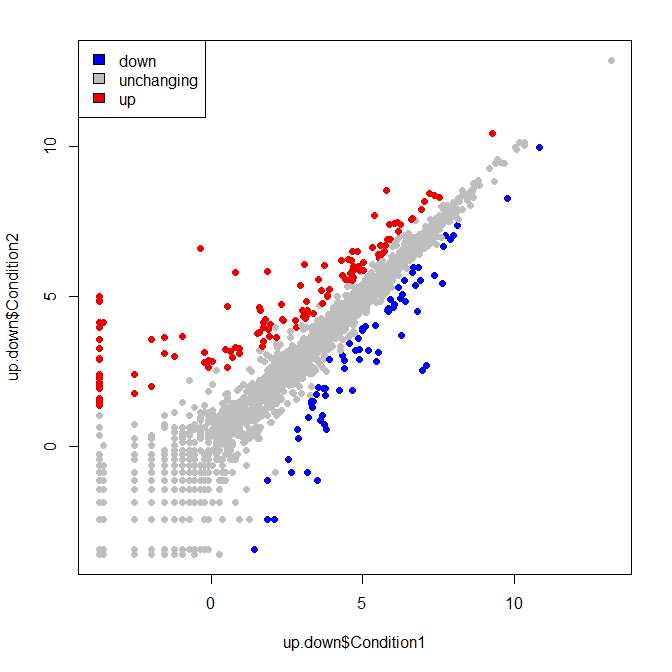Finally we are going to look at the use of quantitative colour. We’re going to use this to plot 3 variables on a single plot.

We can load the data first.

``````read.delim("expression_methylation.txt") -> expr.meth
expr.meth``````